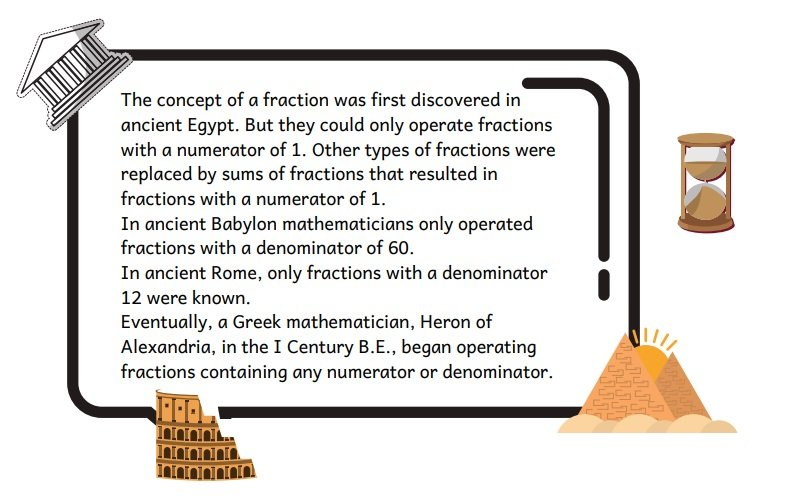# Fractions and Rational ExpressionsFractions and rational expressions are a fun component of math, despite the fact that many students often fear fractions due to lack of understanding of how they work.

Fractions were first discovered in Ancient Egypt. However, the way we represent fractions today came from the Hindus. They were not using the fraction line (bar), though.

Arabic mathematicians started adding the line (bar) that looked like a modern day slash: 1/2. The Lowest Common Denominator concept did not appear until the 16th century. And a common denominator was found when dividing fractions for a long time, before the reciprocal of a fraction was introduced.

There are so many ways to demonstrate the relationships between fractions and wholes. Teachers use manipulatives, real life examples, songs, mnemonics and different visuals. It definitely pays off and students are able to quickly compare fractions represented as Lego pieces or pizza slices and do basic operations with them.

Where students eventually lose confidence, though, is when visuals and manipulatives turn into algebra on paper/tablet and when they need to go further, working with rational expressions that involve variables.

Algebraic representations of fractions and rational expressions are an integral part of many complex computations. It is helpful to be able to operate fractions and rational expressions comfortably on paper and recognize the patterns within equations, word problems involving rates and when working with functions.

In this blog post we are offering activities on fractions and rational expressions for different ages and abilities. They will help achieve the necessary instructional balance between learning using manipulatives and applying skills to solving algebra problems in pencil. The activities also include some interesting facts about the origin of fractions and special cases involving fractions.Rational Expressions Worksheet

For video lessons on fractions, notes and additional practice check out IntoMath.

0